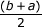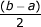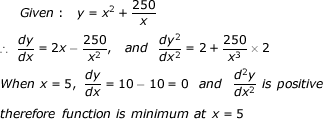# Calculus - Calculus MCQ

41:

The value of ξ in the mean value theorem of
f(b) - f(a) = (b - a)f' (ξ)
for f(x) = Ax2 + bx + C in (a, b) is

 A. b + a B. b - a C.D.Answer Report Discuss Option: C Explanation :Click on Discuss to view users comments. Write your comments here:
42:

The function, y = x2 + 250 / x = 5 at x = 5

 A. maxima B. minima C. maxima nor minima D. 1 Answer Report Discuss Option: B Explanation :Click on Discuss to view users comments. Write your comments here:
43:

If a function is continuous at a point, then its first derivative

 A. may or may not exist B. exists always C. will not exist D. has a unique value Answer Report Discuss Option: D Explanation : Click on Discuss to view users comments. Write your comments here: